UIHistories Project: A History of the University of Illinois by Kalev Leetaru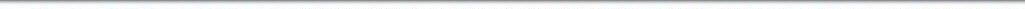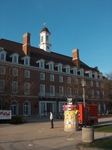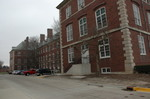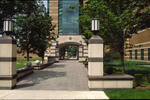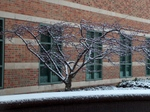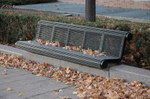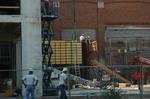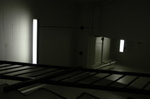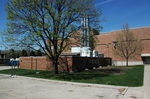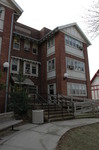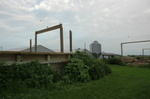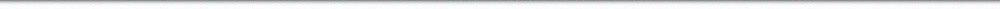N A V I G A T I O N D I G I T A L L I B R A R Y

## Repository: UIHistories Project: Book - Century of Physics (1973) [PAGE 22]

Caption: Book - Century of Physics (1973)
This is a reduced-resolution page image for fast online browsing.

< Previous Page [Displaying Page 22 of 39] Next Page >
[VIEW ALL PAGE THUMBNAILS]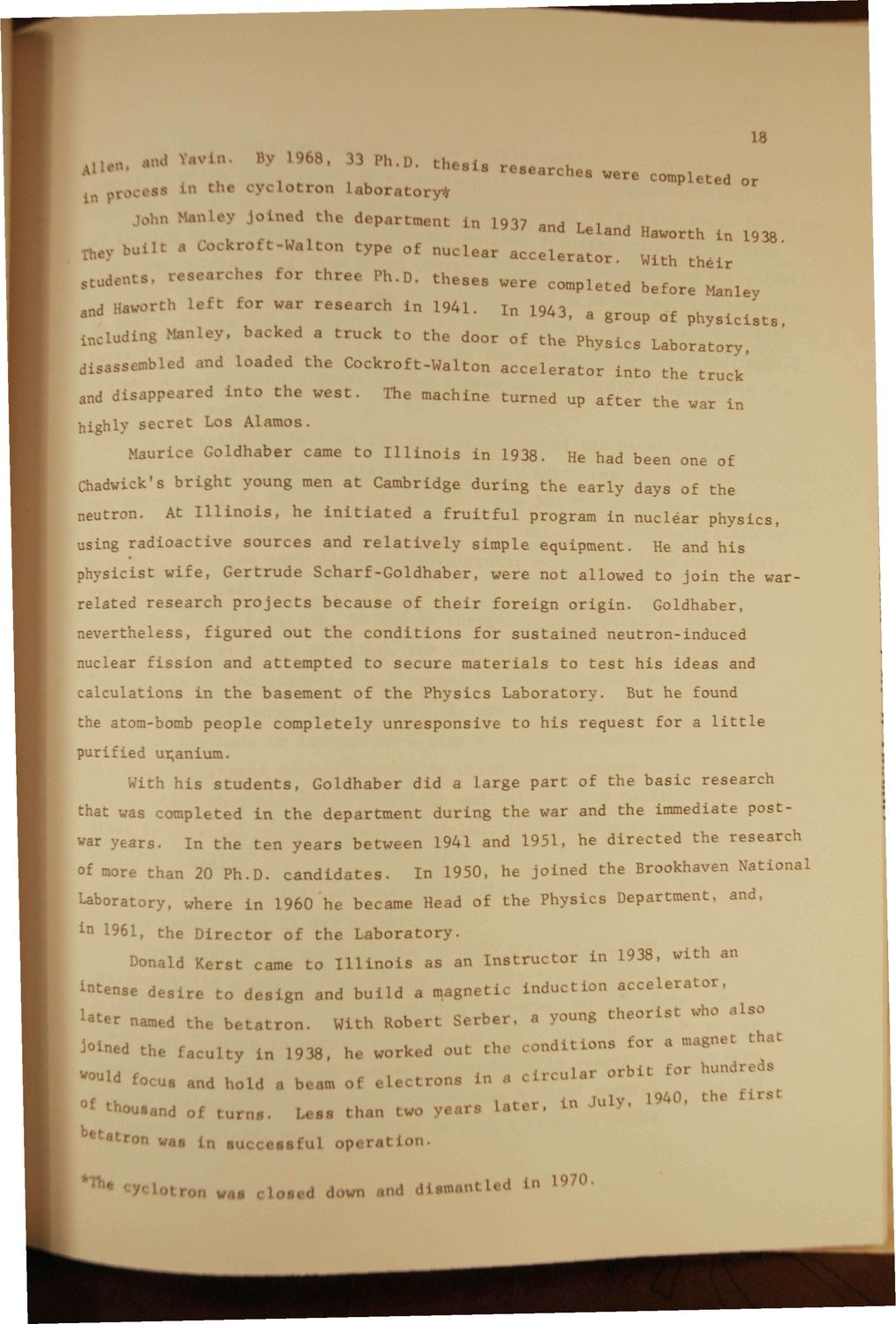### EXTRACTED TEXT FROM PAGE:

18

„ H

tnd V.nvin.

e8S

B) l->68.

I Ph.D. t h t . l , laboratory*

Ln the c y c l o t r o n

research.. r e s e a r c h e s were completed nr

in 1917 a„A i i »37 and Leland llaworth in 1938 jhev built a Oockroft-Waltor, type of n u c l e a r a c c e l e r a t o r . With t h e i r . cos, Che. * t h r e e Ph.D. t h e s e s were completed b e " f o r e " Z l e y iKl,,

and

John M.inl.

J o i n e d t h e department

Haworth I

c for war r e s e a r c h in 1941.

In

m 3 >

.

group

Qf

physicists

including

lov. backed a t r u c k t o t h e door of t h e Physics Laboratory, The machine turned up a f t e r the war in

disassembled and loaded t h e Cockroft-Walton a c c e l e r a t o r i n t o the t r u c k ' and disappeared i n t o t h e w e s t . highb" s e c r e t Los Alamos. Maurice Goldhaber came t o I l l i n o i s in 1938. neutron. At I l l i n o i s , he i n i t i a t e d a f r u i t f u l He had been one of

Chadwick's b r i g h t young men a t Cambridge d u r i n g t h e e a r l y days of the program in nuclear p h y s i c s , He and h i s Goldhaber, using r a d i o a c t i v e s o u r c e s and r e l a t i v e l y s i m p l e equipment. physicist w i f e , G e r t r u d e S c h a r f - G o l d h a b e r , related r e s e a r c h p r o j e c t s b e c a u s e of t h e i r f o r e i g n o r i g i n .

were not allowed to j o i n the war-

nevertheless, f i g u r e d o u t t h e c o n d i t i o n s for s u s t a i n e d neutron-induced nuclear f i s s i o n and a t t e m p t e d t o s e c u r e m a t e r i a l s to t e s t h i s ideas and c a l c u l a t i o n s in t h e basement of t h e P h y s i c s L a b o r a t o r y . purified uranium. With h i s s t u d e n t s , Goldhaber did a l a r g e p a r t of the b a s i c research that was completed in t h e d e p a r t m e n t d u r i n g t h e war and the immediate postwar years. In t h e t e n y e a r s between 1941 and 1951. he d i r e c t e d the research In 1950, he j o i n e d the Brookhaven National But he found the atom-bomb p e o p l e c o m p l e t e l y u n r e s p o n s i v e t o h i s r e q u e s t for a l i t t l e

of more :han 20 Ph.D. c a n d i d a t e s .

Laboratory, where i n 1960 he became Head of t h e Physics Department, and, 1961, the D i r e c t o r of t h e L a b o r a t o r y . Donald Kerst came t o I l l i n o i s as an I n s t r u c t o r in 1938, with an

*tenae deeir< to design and build a magnetic Lnduct Lon accelerator,

U t e r named t h e b e t a t r o t J^ned the fac

J 0 eta

With Robert S e r b e r . a young t h e o r i s t who a l s o

y in 1938, he worl J out the c o n d i t i o n s for a magnet that

oU turni a

<fo

mmH

beam of e.

I one L a circular orbit cor hundreds n

u-n

.

Leas than two y n r . Later, in July, 1940. the Eire!

>** Ln succeasiui operation. loti waa d > n .'mil diemanl led Ln L970. w

*